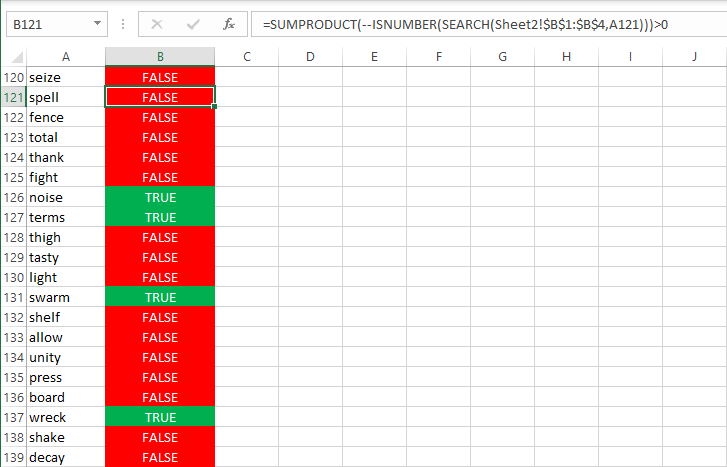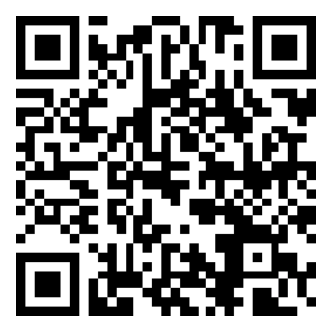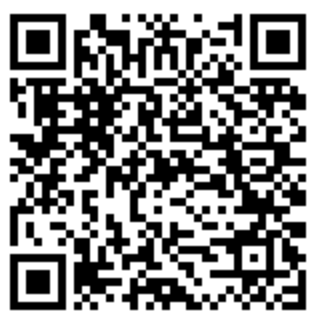# Excel - Check a column for values found in another column

What?
This is an article to remind me how to search a column in an Excel file for values found in another column (in this example, on another worksheet in the same workbook).

How?
So for demonstration purposes, I'm using a new Excel file with two worksheets called "Sheet1" and "Sheet2" respectively.

Sheet1 contains the following:
```A               B
--------------- ---------------
seize
spell
fence
total
thank
fight
noise
terms
thigh
tasty
light
swarm
shelf
allow
unity
press
board
wreck
shake
decay
```
Sheet2 contains the following::
```A
---------------
warm
noise
term
wreck
```

The formula to paste in column B of Sheet1 is as follows:
```=SUMPRODUCT(--ISNUMBER(SEARCH(Sheet2!\$A\$1:\$A\$4,Sheet1!A1)))>0
```
Where:
• SumProduct( is a function to multiply two ranges together (must be the same size) and return the total of these results.
• -- is a double hyphen/dash which converts a boolean true to 1 and boolean false to 0.
• IsNumber( is a function returning a boolean. Returns false if a cell value has been formatted to a text type. Returns true if the cell value is a number AND the cell data type is numeric.
• Search( is a case-insensitive search with the first parameter being the text to search for (can be a range) and the second the string to search (in this case a single cell value).
• Sheet2!\$A\$1:\$A\$4 is the range of words to find from Sheet2. Note that there is a dollar in front of both column name (eg. "A") and the row number (eg. "1") so that these don't automatically change when you copy the formula to other parts of the spreadsheet (ie. not relative).
• Sheet1!A1 is the cell to search for those words. Note how I don't add a dollar, this is so that the formula will apply to each row and increment based on how you copy the formula to other cells.

You should end up with something like this (I have added some conditional formatting which colors the cells red or green for demonstration purposes):If you understand this article, you may have noticed that the range of words to find from Sheet2 in this screenshot are from the cells B1:B4... This is because I already had values in A1:A4 and wanted to test against a set of different words.

### Accreditation### Donate & Support

If you like my content, and would like to support this sharing site, feel free to donate using a method below:

Paypal:Bitcoin:3QnhmaBX7LQSRsC9hh6Je9rGQKEGNQNfPb
© 2021 Joel Lipman .com. All Rights Reserved.